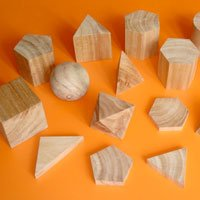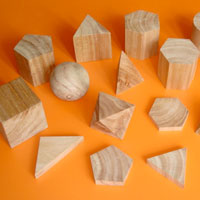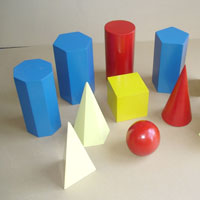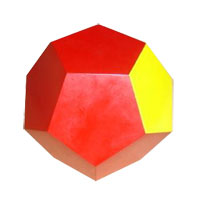Kumar Instruments Co.

Ambala, Haryana, India

# Mathematical Shape ModelsThe company is a major supplier of Mathematical Shape Models based in India. We offer Mathematical Shape Models that are specially designed in order to enhance the learning power in children. These Mathematical Shape Models are designed interestingly, in order to make the learning experience a fun filled one. We offer different types of Mathematical Shape Models that can help children study volume of cylinder, give more knowledge about cube, cuboids etc. The clients can avail these Mathematical Shape Models at very affordable prices from us.

To enquire about the desired product(s), just check the box and then click â��Inquiry Nowâ�� button which is provided below.

## Products Images

Image Details• Model No M-52

We are a renowned Mathematical Shape Models (Model No M-52) Supplier in India. The Mathematical Shape Models (Model No M-52), offered by us, are 3 dimensional with smooth finish. Our Mathematical Shape Models (Model No M-52) can be availed in minimum set of 16 in plastic transparent container. Avail the Mathematical Shape Models (Model No M-52) from us at affordable prices.

Size Measuring : 40 mm• Model No M-53

We are engaged in offering Mathematical Shape Models (Model No M-53) which are 3 dimensional in shape for precise understanding. Made using medium density fiber construction, our Mathematical Shape Models (Model No M-53) features colored finish. The size measuring of Mathematical Shape Models (Model No M-53) is 75 x 150 mm and can be availed in minimum set of 12.• Model No M-54

Geometrical model we are offering 3 dimensional Mathematical Shape Models (Model No M-54). Our Mathematical Shape Models (Model No M-54) feature colored medium density fiber board construction and 12 regular pentagonal faces. Our Mathematical Shape Models (Model No M-54) appear to be an art of perfection. We are a trusted Mathematical Shape Models (Model No M-54)

Looking for Mathematical Shape Models?